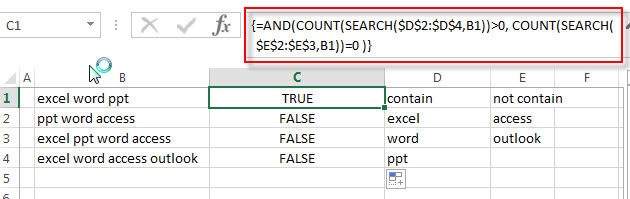# Check if Cell Contains Certain Values but do not Contain Others Values

In the previous post, we only talked that how to check a cell if contains one of several values from a range in excel. And this post explains that how to check a cell if it contains certain values or contains one of several values but do not contain other certain values in another range or a list in excel.

## Check if Cell Contains Certain Values but do not Contain Others Values

Supposing that you have a list of text strings in the range B1:B3 and you need to check each Cell that if it contains one of several values in a range D2:D4 but do not contains any of values in range E2:E3.  If TRUE, then returns TRUE value, otherwise, returns FALSE value.

To check a cell to see if it contains certain string but do not contain others. You can use a combination of the AND function, the COUNT function and the SEARCH function to create a new array formula as follows:

`=AND(COUNT(SEARCH(\$D\$2:\$D\$4,B1))>0, COUNT(SEARCH(\$E\$2:\$E\$3,B1))=0 )`

Let’s see how this formula works:

= SEARCH(\$D\$2:\$D\$4,B1)

The SEACH function returns position of the first character of find_text in a text string. And this formula will search each value in the range D2:D4 inside within_text in Cell B1, then returns position of each text string in Cell B1, so it will return an array result like this:

`{1;7;12}`

= SEARCH(\$E\$2:\$E\$3,B1)

This SEARCH formula will search each value in range E2:E3 inside within_text in Cell B1, and then returns position of the first position of each find_text in Cell B1. When no match is found, the SEARCH function will return the #VALUE error. So it will return an array result like this:

`{#VALUE!;#VALUE!;1}`

=COUNT(SEARCH(\$D\$2:\$D\$4,B1))>0

The COUNT function will count the number of cells that contain numbers in an array returned by the SEARCH function. If it is greater than 0, then it indicated that at least one values is found in Cell B1.

=COUNT(SEARCH(\$E\$2:\$E\$4,B1))=0

If the number of cells that contain numbers is equal to 0, then it means that none of values can be found in Cell B1.

=AND(COUNT(SEARCH(\$D\$2:\$D\$4,B1))>0, COUNT(SEARCH(\$E\$2:\$E\$4,B1))=0 )

If both two COUNT function return TRUE value, the AND function returns TRUE. And if either COUNT function is FALSE, this formula returns FALSE.### Related Functions

• Excel SEARCH function
The Excel SEARCH function returns the number of the starting location of a substring in a text string.The syntax of the SEARCH function is as below:= SEARCH  (find_text, within_text,[start_num])…
• Excel COUNT function
The Excel COUNT function counts the number of cells that contain numbers, and counts numbers within the list of arguments. It returns a numeric value that indicate the number of cells that contain numbers in a range…
• Excel AND function
The Excel AND function returns TRUE if all of arguments are TRUE, and it returns FALSE if any of arguments are FALSE.The syntax of the AND function is as below:= AND (condition1,[condition2],…)…

Related Posts

Filter or Extract with a Partial Match

Assume you have a table consisting of a few cells having few values, and you want to filter out the set of records with the partial match. You might take it easy and would prefer to manually filter out the ...

Extract all Partial Matches

Just assume that you have a range of data that contains a text string and you want to extract the few partial matches into another separate range of cells; then you might think that it's not a big deal; because ...

If Cell Contains Certain Text OR Equals Certain Text

IF cell equals certain text IF function is frequently used in Excel worksheet to return “true value” or “false value” based on the logical test result. If you want to test values to see if they equal certain text like ...

How to Extract Text between Two Text Strings in Excel

This post will guide you how to extract text between two given text strings in Excel. How do I get text string between two words with a formula in Excel. Extract Text between Two Text Strings Assuming that you have ...

How to Return a Value If a Cell Contains a Specific Text in Excel

This post will guide you how to return a value if a cell contains a certain number or text string in Excel. How do I check if a Cell contains specific text and then return another specific text in another ...

Insert The File Path and Filename into Cell

This post will guide you how to insert the file path and filename into a cell in Excel. Or how to add a file path only in a specified cell with a formula in Excel. Or how to add the ...

Sort Cells by Specific word or words

This post will guide you how to sort cells or text values in a column based on a specific word even if the word is in the text string in the cell. How do I sort cells in a column ...

Highlight Rows

This post will teach you how to highlight rows in a table with conditional formatting in Excel. You will learn that how to change the color of the entire rows if the value of cells in a specified column meets ...

How to replace all characters after the first specific character

This post will guide you how to replace all characters after the first match of a specific character with a new text string in excel. How to replace all substrings after the first occurrence of the comma character with another ...

How to extract text after the second or nth specific character (space or comma)

In the previous post, we talked that how to extract text after the first occurrence of the comma character in excel. And this post explains that how to get a substring after the second or nth occurrence of the comma ...

Sidebar